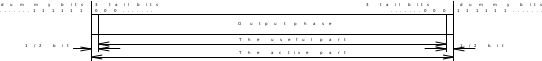## 2 Modulation format for GMSK

05.043GPPModulationTS

## 2.1 Modulating symbol rate

The modulating symbol rate is 1/T = 1 625/6 ksymb/s (i.e. approximately 270.833 ksymb/s), which corresponds to 1 625/6 kbit/s (i.e. 270.833 kbit/s).

## 2.2 Start and stop of the burst

Before the first bit of the bursts as defined in GSM 05.02  enters the modulator, the modulator has an internal state as if a modulating bit stream consisting of consecutive ones (di = 1) had entered the differential encoder. Also after the last bit of the time slot, the modulator has an internal state as if a modulating bit stream consisting of consecutive ones (di = 1) had continued to enter the differential encoder. These bits are called dummy bits and define the start and the stop of the active and the useful part of the burst as illustrated in figure 1. Nothing is specified about the actual phase of the modulator output signal outside the useful part of the burst.Figure 1: Relation between active part of burst, tail bits and dummy bits.
For the normal burst the useful part lasts for 147 modulating bits

## 2.3 Differential encoding

Each data value di = [0,1] is differentially encoded. The output of the differential encoder is:

where  denotes modulo 2 addition.

The modulating data value i input to the modulator is:

## 2.4 Filtering

The modulating data values i as represented by Dirac pulses excite a linear filter with impulse response defined by:

where the function rect(x) is defined by:

and * means convolution. h(t) is defined by:

where

where B is the 3 dB bandwidth of the filter with impulse response h(t), and T is the duration of one input data bit. This theoretical filter is associated with tolerances defined in GSM 05.05 .

## 2.5 Output phase

The phase of the modulated signal is:

where the modulating index h is 1/2 (maximum phase change in radians is /2 per data interval).

The time reference t’ = 0 is the start of the active part of the burst as shown in figure 1. This is also the start of the bit period of bit number 0 (the first tail bit) as defined in GSM 05.02 .

## 2.6 Modulation

The modulated RF carrier, except for start and stop of the TDMA burst may therefore be expressed as:

where Ec is the energy per modulating bit, f0 is the centre frequency and 0 is a random phase and is constant during one burst.